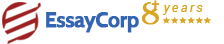WhatsApp
Struggling With Assignments?

We are here to help.

Chat with our expert writers on WhatsApp

for any kind of assignment queries.

Click to chat!
Struggling with Assignments? WhatsApp Us### Question :

Conduct a simple linear regression analysis to examine the relationship between ‘education’ (the independent variable) and ‘wage’ (the dependent variable). Using the Excel data file, prepare a 2000 word report using the following structure.

#### BACKGROUND AND PURPOSE

In statistics, regression analysis is a statistical process for estimating the relationships among variables. It includes many techniques for modeling and analyzing several variables, when the focus is on the relationship between a dependent variable and one or more independent variables.

Let X1, X2,..., Xp be p independent variables and Y be some variable dependent on X1, X2, ... , Xp . The basic idea in regression analysis is to explore a functional relationship between Y and X1, X2, ... , Xp , i.e.

`Y = f(X1, X2, ... , Xp)`

For simplicity, we use a class of functions where Y and X that are related through a linear function of some unknown parameters which leads to linear regression analysis. Let f takes the following form,

`Y = β0 + β1X + ε`

Above equation specifies what we call as simple linear regression model (SLRM) where β0, β1 are termed as regression coefficients. We are interested in estimating the unknown parameters β0, β1on the basis of a random sample from Y and given values of the independent variable.

### METHOD

We perform linear regression analysis by using the "ordinary least squares" method to fit a line through a set of observations. We can analyze how a single dependent variable is affected by the values of one or more explanatory variables. For example, we can analyze the number of deaths due to cigarette smoking or lung cancer or in our case we can analyze how number of years of education affects the hourly wages. Suppose we have one variable model with regression equation as Yi = α + β Xi + εi. the subscript i denotes observations. So that, we have (y1, x1), (y2, x2), etc. The εi term is the error term, which is the difference between the effect of xi and the observed value of yi. The OLS works on the principle of minimizing the sum of squares more specifically the squared error terms. We need to minimize the 2 variables with respect to α and ß. Thus we have:

### SIMPLE LINEAR REGRESSION ANALYSIS

To examine the relationship between ‘education’ (the independent variable) and ‘wage’ (the dependent variable), we examine:

#### a) Summary statistics and histograms:

From the summary statistics we can infer that the mean wage and mean education years are 22.31 13.76, means that their respective data is clustered around these averages. Also the standard deviation of wage 14.02 describes high dispersion in data from the mean value and the standard deviation of education 2.73 describes lesser dispersion in data from the mean value. This means the data for education is much closely packed as compared to the wages. Skewness of wage approx 1.5 which tells that the data might be positively skewed whereas skewness for education is approx 0 which means data might be symmetrical. Also the kurtosis of both wage and education tell that curve will be platykurtic in nature.

HIRE EXPERT
Email ID *
Subject
Pages